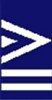SimCon - Fortran Analysis, Engineering & Migration | FPT Reference Manual | Downloads | Licensing | Home
 Research Fortran Tools
 Checking Fortran Expressions Checking Expressions WinFPT and FPT check expressions for inconsistencies. The checks include: Loss of Precision This occurs, for example, when a REAL variable receives a DOUBLE PRECISION value as in: REAL*8 theta REAL*4 x : x = 100.0D0*COS(theta) !---------------------------^-------------------------------------------------- !!! FPT - 3067 Loss of precision in real or complex assignment !------------------------------------------------------------------------------ or when a high precision variable receives a lower precision result, and therefore does not have the expected precision: REAL*8 d REAL*4 x,y : d=SQRT(x**2+y**2) !----------------------^------------------------------------------------------- !!! FPT - 3069 The left-hand-side variable will not have the expected precision !------------------------------------------------------------------------------   Risk of Overflow This occurs when an INTEGER variable receives a value from a REAL expression or from an INTEGER expression with a larger data size. For example: INTEGER*2 i2 INTEGER*4 j REAL x : i2=j+1 !---------^-------------------------------------------------------------------- !!! FPT - 3073 Danger of overflow due to different integer sizes !------------------------------------------------------------------------------ : i2=x !---------^-------------------------------------------------------------------- !!! FPT - 2865 REAL value assigned to INTEGER variable - danger of overflow !------------------------------------------------------------------------------   Integer Quotients This occurs when an INTEGER division is converted to a REAL result, either across an equals sign or within an expression. Often (as below) the intent is to produce a REAL value from the division. approx_error = 22/7-4.0*ATAN(1.0) !--------------------------------------^--------------------------------------- !!! FPT - 3079 Integer result of an integer division coerced to a real value !------------------------------------------------------------------------------ In this case, the sub-expression 4.0*ATAN(1.0) is a common trick to generate the value of PI. 22/7 is a favourite school child's approximation for PI, and the intent was to find the error. However, the sub-expression 22/7 contains only integer terms and is computed as the integer 3, not as the real value 3.14286.   Integer Negative Powers The result of raising an INTEGER to a negative integer power is always zero unless the original integer value is 1. For example, the value    I ** (-2) is computed as:    1 / (I**2) which is performed as an integer division. I**2 will be larger than 1 unless I has a value of 1, so the result is usually zero. WinFPT and FPT trap this, for example: x = npri**(-3) !-------------------^---------------------------------------------------------- !!! FPT - 3075 Integer raised to negative power - The result is usually zero !------------------------------------------------------------------------------ We have found an example of this problem in a real-time control system.   Exact Comparison of REAL or COMPLEX variables Testing REAL or COMPLEX values for exact equality is, at best, unreliable, and is not portable because of variations in machine precision. For example: IF (x .EQ. 1.0) THEN !----------------^------------------------------------------------------------- !!! FPT - 3087 REAL or COMPLEX quantity tested for exact equality/inequality !------------------------------------------------------------------------------ A far less common variant (which we have encountered in aircraft design code) is: REAL*8 p : IF (p)200,220,240 !----------^------------------------------------------------------------------- !!! FPT - 3061 Arithmetic IF operand is of type REAL !------------------------------------------------------------------------------ The second destination label is used when (if ever!) the REAL argument of the IF is exactly zero.   REAL Variables used as Array Indices The danger in this case is that the REAL value will be very slightly below the corresponding integer. The array index will then be one less than was intended. REAL*8 masses(100,100),sm REAL*4 p : sm = sm+masses(i,p) !------------------------^----------------------------------------------------- !!! FPT - 3103 Array index is of type REAL !------------------------------------------------------------------------------   Character String Truncation WinFPT / FPT warns of possible data loss: CHARACTER*128 1 userfile 1 , fullfile : IF (userfile(2:2) .NE. ':') THEN fullfile = 'C:'//userfile !---------------------------------^-------------------------------------------- !!! FPT - 1253 String is truncated on assignment !------------------------------------------------------------------------------   Integer used as Logical Some compilers allow INTEGER variables to be used in LOGICAL contexts. Of these, some use the least significant bit as the flag, some use non-zero as .TRUE. and some use negative as .TRUE.. At best, the usage is not portable. It is trapped, for example: INTEGER l3 : LOGICAL l1,l2 : IF (l1 .OR. l2 .OR. l3) THEN !-------------------------^-^-------------------------------------------------- !!! FPT - 2679 .AND. or .OR. used with integer operand(s), result is integer !!! FPT - 2533 Incorrect data type for this IF statement !------------------------------------------------------------------------------